## Department of Computer Science# Gröbner Basis Theory

 Contents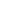Introduction via Lego bricksGröbner Bases and Polynomial IdealsGröbner Basis Theory for RewritersGröbner Bases: Rules and Truths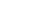Search## Introduction via Lego bricks

A mathematical structure can often be presented in terms of a set of generators with composition and rules (also called relations). In terms of Lego, our generators are the bricks - the building blocks and composition is simply the idea of sticking the blocks together to make Lego-models. Equivalence is illustrated by the idea of two different collections of blocks being used to build Lego-models which appear the same. If the sub-models making up larger models are equivalent then we can deduce that the larger models are equivalent - the rules about small pieces can be applied in combination to larger models.

In some respects, the Lego example is quite deep because many different possible compositions exist, although the example of equivalence is not very nice - since it is not obvious here that the rules about all lego-models can be built up from rules about equivalence of little models... The problems of modelling compositions beyond the scope of sums on a line are addressed in higher dimensional algebra. We will not discuss that here, but you might like to follow the link to Ronnie Brown's page on Higher Dimensional Group Theory for some very interesting ideas and more links.

## Gröbner Bases and Polynomial Ideals

If X is a set, then the free semigroup on X contains all power products of elements of X, with multiplication defined in the usual way and will be denoted X° -- because I'm writing in XML :(. Let K be a field, then K[X°] is the ring of polynomials p = k1 m1 + ··· + kt mt where k1, ..., kt are in K and m1, ..., mt in X° with the operations of polynomial addition and polynomial multiplication defined in the usual way. Consider a subset of polynomials P. The ideal generated by P is denoted <P> and includes all sums u1 p1 + ··· + un pn where u1, ..., un are in K[X°] and p1, ..., pn are in P. A pair of polynomials q and q' are said to be equivalent modulo P, if their difference q-q' is a member <P>. Determining this is a commonly encountered problem, generally known as the ideal memebership problem.

In 1965 Bruno Buchberger invented the concept of a Gröbner basis and developed algorithms which allow us to solve the ideal membership problem. The key ingredient for converting a given set of polynomials into this magical thing called a Gröbner basis, is a well-ordering on the monomials. The well-ordering allows us to define the leading monomial LM(p) of each polynomial p. This will give us a reduction relation on the polynomials. Given a polynomial q we can reduce it if any of its terms contains the leading monomial of a polynomial p in the basis P. The reduction replaces the term in which the leading monomial occurs with a collection of terms, all of which are smaller with respect to the ordering. Formally, we can write q -> q-ku(p)v where ku(LM(p))v occurs in q for some k in K and some monomials u,v in X°.

The key property characterising a Gröbner basis is that the reduction relation it generates is Noetherian and confluent. This means that the ideal membership problem can be solved by reducing the polynomials to their unique normal forms - if they are in the ideal then they will reduce to zero - if they are not, then they won't.

The process of converting a basis into a Gröbner basis is fiddly, even for small examples, and is best achieved using a computer. The principle os to identify all the obstacles to confluence (S-polynomials) and add them in to the basis. Identification is possible because of the fact that every possible conflict is the result of an overlap between two leading monomials. By adding in the difference between the two possible reductions the reduction relation becomes more joined-up, the ordering forces it to become joined up in a smooth, sensible way. The S-polynomials added may of course cause conflict with each other, or with the original polynomials. At each stage the S-polynomials are added; then conflicts must be checked for again. With luck the procedure will terminate - if it does not, it may be the case that using a different ordering will help, or that the S-polynomials can be examined for a pattern, and an infinite Gröbner basis specified.

## Gröbner Basis Theory for Rewriters

String-rewriting is a special case of Gröbner basis theory - where all the polynomials are "difference binomials", that is they have two terms, one with coefficiant -1 and one with coefficient +1. If you know rewriting then think of Gröbner bases this way: "you still have l->r and l is still a string, but now r might be a polynomial". Really there is not so much difference: the Knuth-Bendix algorithm is step-for-step identifiable as the Buchberger algorithm applied to bases for difference binomials.

String-rewriting Theory Gröbner Basis Theory
rewrite system basis
rule two-term polynomial
(difference binomial)
string monomial
reduction reduction
sub-string sub-monomial (divisor)
right hand side remainder
overlap match
critical pair S-polynomial
resolve reduce to zero
reduced critical pair reduced S-polynomial
complete rewrite system Grobner basis

You may be interested in "Rewriting as a Special Case of Gröbner Basis Theory" which contains the results in more detail.

## Gröbner Bases: Rules and Truths

Basically, I am interested in the types of problem which can be expressed as: given a structure described by generators, composition and rules - find out what is true within that structure. My focus is on the rules, combining them to find a new rules and preferably, a collection of rules which will completely describe the structure. Gröbner basis theory is a very powerful tool for tackling this kind of problem whenever the elements one is manipulating look like polynomials.

The main disadvantages are that Gröbner basis computations can use a lot of computer power - and often, if the compositions are not commutative, they will never terminate with a finite rule system. Despite these disadvantages, Gröbner basis theory is impressively powerful, and appears to be a very useful tool in each new field that it is applied. I am interested in applying Gröbner bases in (enriched) category theory, giving them a very general setting and widening their scope. I'm also hopeful of using automata to provide information about and perhaps even an alternative to infinite Gröbner bases.

Next: Applications of Grobner basis theory (under construction).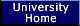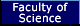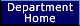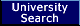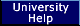Author: Anne Heyworth
Created: 26th April 2001